# Machines Learning to Solve Sign Problemswith Hyunwoo Oh, Yukari Yamauchi, Andrei Alexandru, Paulo Bedaque, Henry Lamm, Neill Warrington
September 5, 2022 at אוניברסיטת תל אביב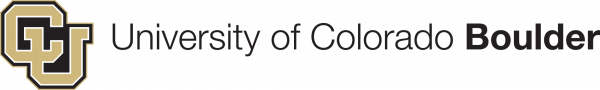# The Easiest Sign Problem

$Z = \int d x\; e^{-x^2 - {\color{red}2 i \alpha x}}$ $\color{blue} Z_Q = \int d x \; e^{-x^2}$

We must sample with respect to the quenched Boltzmann factor. Observables are computed via $\langle \mathcal O \rangle = \frac{\langle\mathcal O e^{-i S_I} \rangle_Q}{\langle e^{-i S_I}\rangle_Q}$

The sign problem is measured by $\langle \sigma\rangle \equiv \frac Z {Z_Q} \sim e^{-\alpha^2}$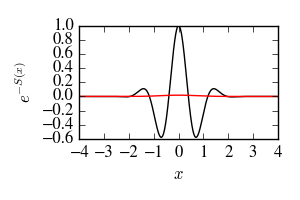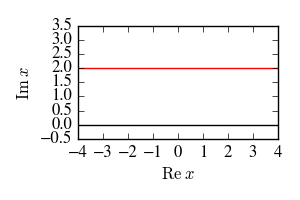# Contour Integrals for the Sign Problem

The Boltzmann factor $$e^{-S}$$ is complex. Sample with $$e^{-S_R}$$ and reweight. $\langle\sigma\rangle = \frac{\int e^{-S}}{\int |e^{-S}|}$Theorem: the integral of a holomorphic function is unchanged by contour deformation.

# Thirring Model

Relativistic fermions in $$1+1$$ dimensions with a repulsive 2-body interaction. $S_{\mathrm{thirring}} = \frac 1 {2 g^2}\sum_{x,\mu} \big(1 - \cos A_\mu(x)\big)- \frac{N_f}{2} \log \det K[A]$

The $$N_f=1$$ theory is exactly solvable. Strong coupling marked by $$m_B \sim m_F$$.

Sign problem exponentially bad in $$m^2 V$$.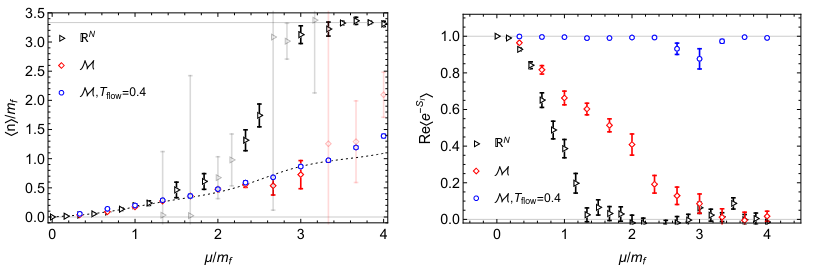# Finding Contours: Holomorphic Gradient Flow

Evolve every point on the real plane according to the holomorphic gradient flow: $\frac{d z}{dt} = \left({\frac{\partial S}{\partial z}}\right)^*$ For short flow times, improves the average sign by decreasing the quenched partition function. (Maximally efficient!) $Z_Q = \int dz\, |e^{-S}|$

Alas, evolving this ODE at every sample is dreadfully slow.

For an $$8^4$$ QCD lattice, evaluating the Jacobian determinant requires a $$10^5 \times 10^5$$ matrix. ($$\sim 10$$ times larger than the Dirac matrix)# Learning Flowed Contours

Algorithm:

• Sample many points from flowed manifold.
• Train neural network to approximate flow (supervised learning)
• Run Monte Carlo using approximated flow.
$\mathop{\mathrm{Re}} A = M_3 \sigma[M_2 \sigma(M_1 \mathop{\mathrm{Im}} A)]$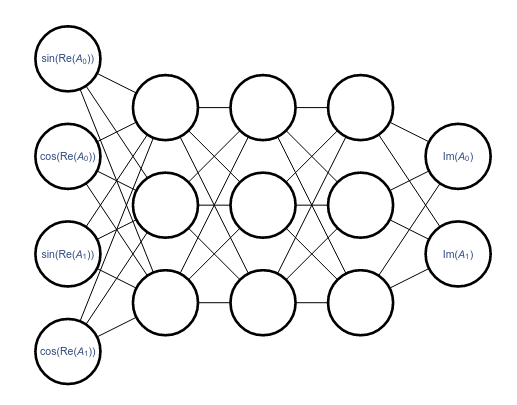Downsides:

• Determinant computation still required.
• Many samples points required to train accurately.
• Flowed manifold is not optimal!

# Direct Contour Optimization

Evaluating $$\langle \sigma \rangle$$ itself is hard. The sign problem manifests as a signal-to-noise problem.

But, we don't need to! We just need to minimize $$Z_Q$$, and $\color{green} \frac{d}{d t}\lambda = - \frac{\partial}{\partial \lambda} \log Z_Q$ has the form of a quenched (i.e., sign-free) observable!

This isn't complex analysis---it's a general principle. Contour deformations:

• Leave $$Z$$ alone, while
• Potentially modifying $$Z_Q$$.

Any strategy with those properties can be optimized in the same way.

# Early Success: Thirring Model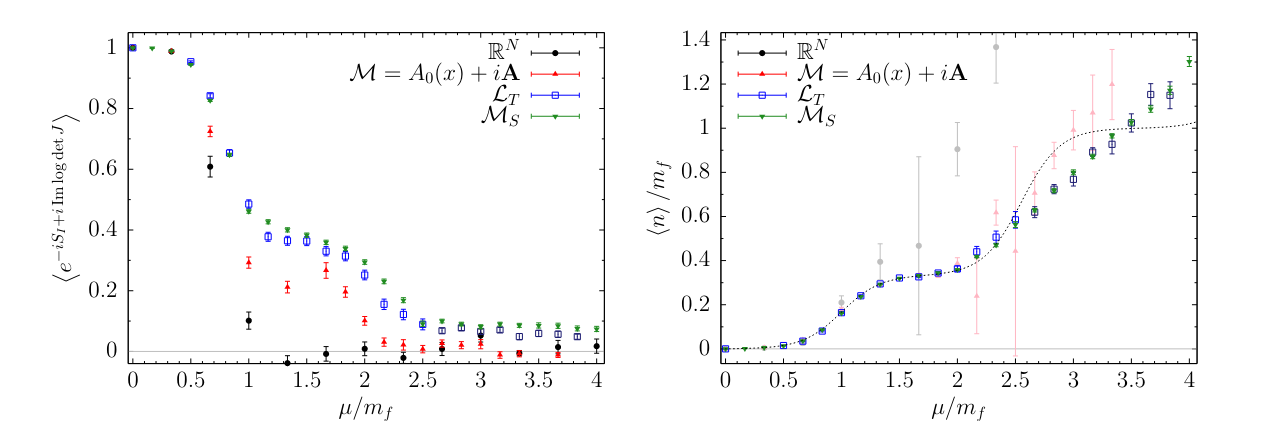Best results obtained with a simple ansatz, rather than a deep neural network: $\mathop{\mathrm{Im}} A_0(x) = a + b \cos \mathop{\mathrm{Re}} A_0(x) + c \cos \mathop{\mathrm{Re}} 2 A_0(x)$

# Another Perspective: Normalizing Flows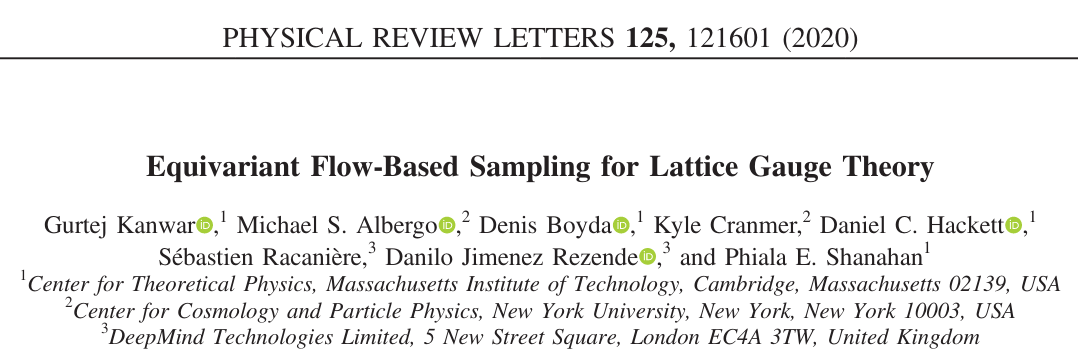Goal: sample from $$p(z)$$

A normalizing flow is a map $$\phi$$ $\int_{-\infty}^x e^{-x^2} dx = \int_{-\infty}^{\phi(x)} p(z)\, dz$

Sample from the Gaussian, then apply $$\phi$$.

(Any easily sampled distribution can replace the Gaussian.)

What if $$\phi(x)$$ is complex?

Complex normalizing flow $$\Rightarrow$$ contour deformation

A complex normalizing flow gives a locally perfect contour deformation

# Analytic Continuation of Normalizing Flows

Here's a normalizing flow for scalar field theory: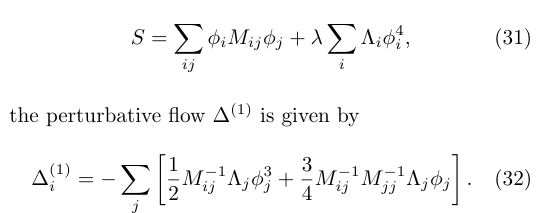What if $$M,\Lambda$$ are complex? Doesn't matter; still a good normalizing flow.

Normalizing flows are analytic in action parameters

To summarize:
• Normalizing flows always exist for real distributions (theorem)
• These can be analytically continued for complex parameters (ideology)
• Complex normalizing flow gives (locally perfect) integration contour

# Scalar Field Theory at Complex Coupling

$S = \int dx\, \frac 1 2 \left(\frac{\partial\phi}{\partial x}\right)^2 + \frac {m^2}{2} \phi(x)^2 + \lambda \phi(x)^4$

At $$\mathop{\mathrm{Im}}\lambda \ne 0$$, this model has a sign problem. Train a contour as before, and the sign problem nearly disappears: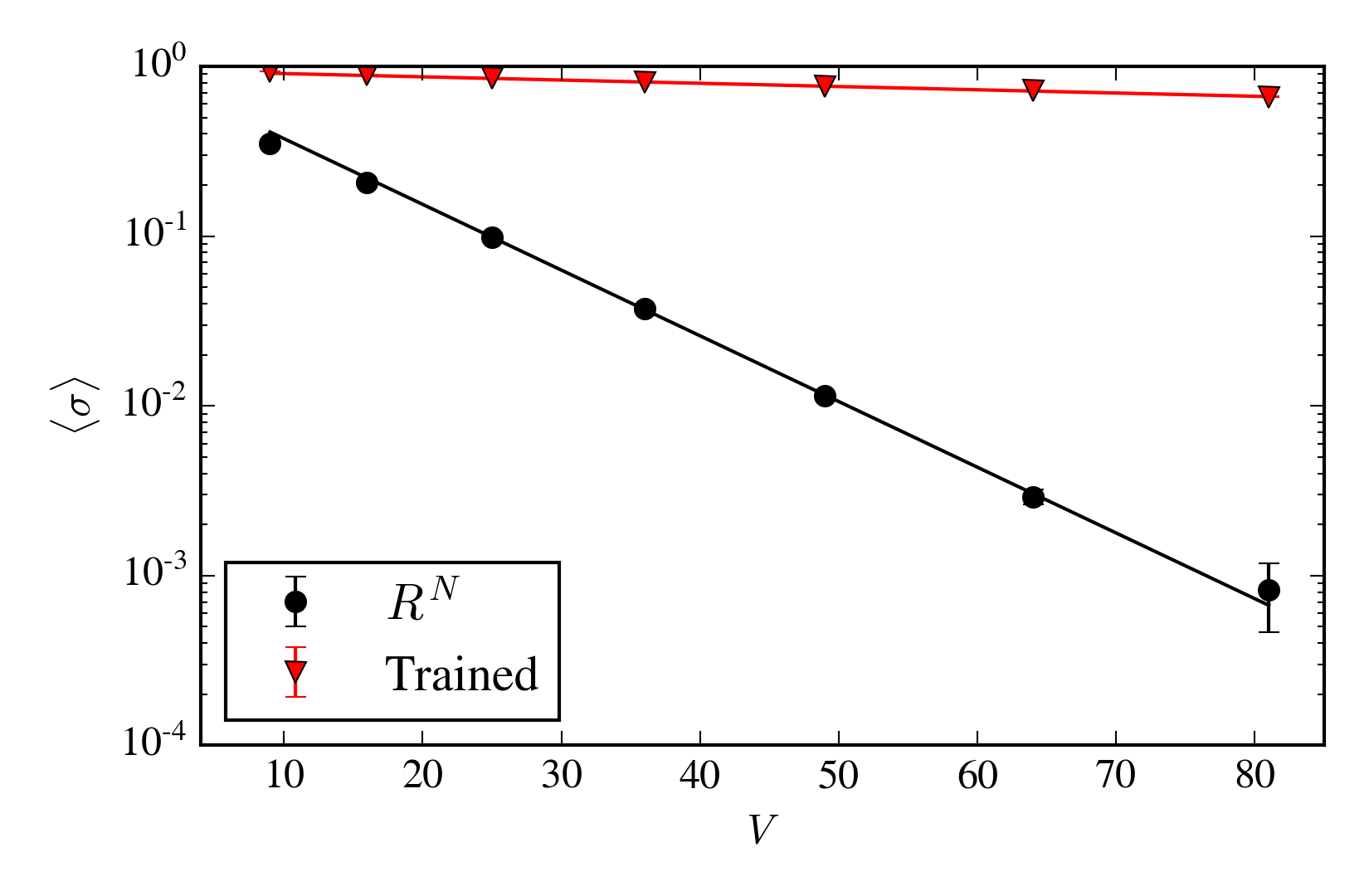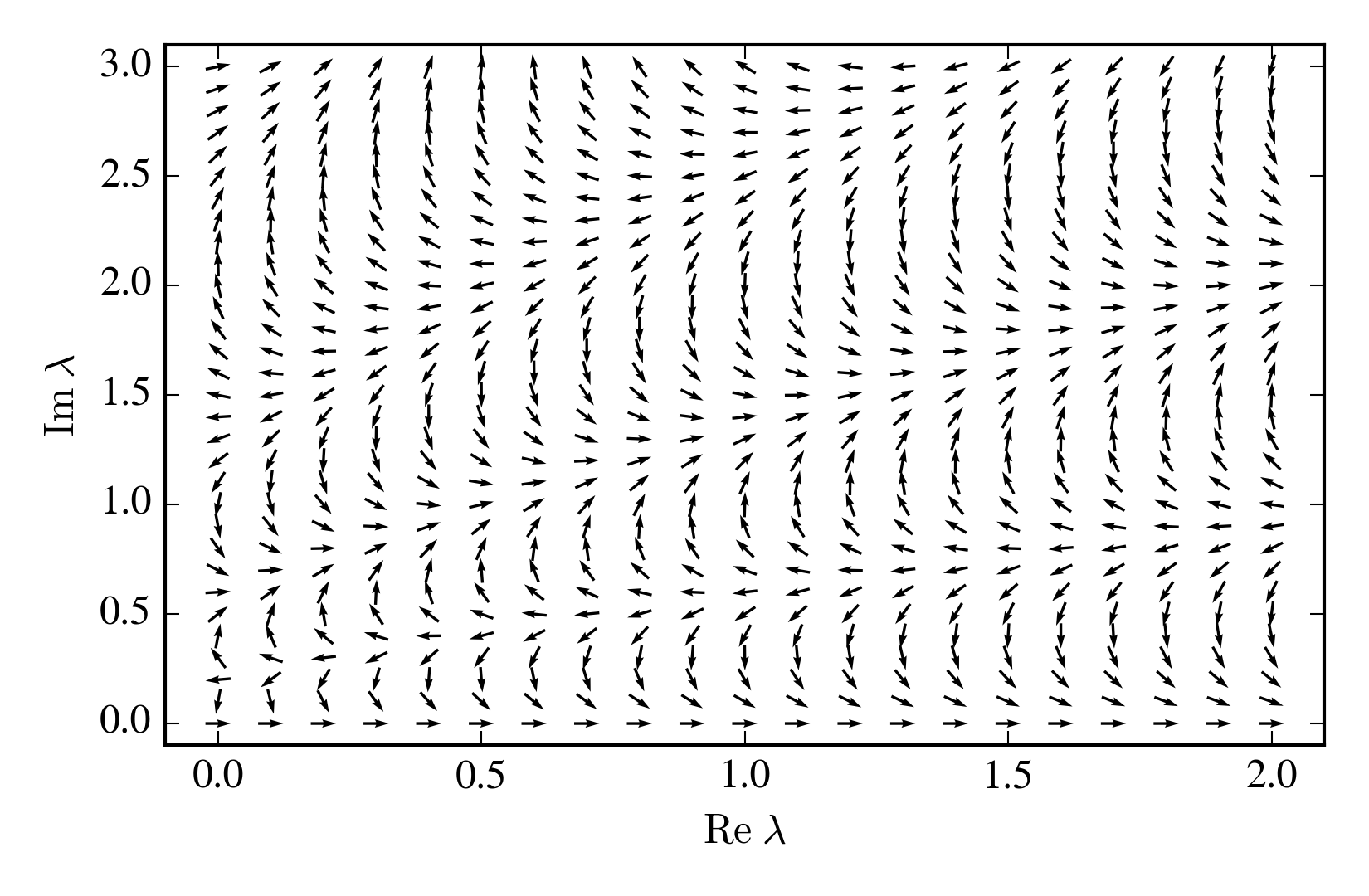# Cost Function for Normalizing Flows

\begin{align} C_{\mathrm{nf}}(\lambda) &= \Bigg\langle\bigg|\underbrace{\frac{\phi^2}{2} - \Re\log\mathcal N - \Re S(\tilde\phi_\lambda(\phi)) - \Re \log \frac{\partial\tilde\phi_\lambda}{\partial\phi}}_{\Re (S_{\mathrm{induced}} - S)}\bigg|^2\Bigg\rangle_n\\ &+ \Bigg\langle\bigg|1-\underbrace{\Big(\mathop{\mathrm{csgn}} \frac{\partial \tilde\phi_\lambda}{\partial \phi}\Big)e^{-i\Im \left(S(\tilde\phi_\lambda(\phi)) + \Im \log \mathcal N\right)}}_{e^{-i \Im \left(S_{\mathrm{induced}} - S\right)}}\bigg|^2\Bigg\rangle_n \text. \end{align}
Adiabatic training: Start at real $$\lambda$$ and rotate $$\lambda$$ while tracking a "good" contour (or normalizing flow).

# Analytically Continued Scalar Field Theory

$Z(\lambda) = \int \mathcal D\phi\, e^{- (\cdots + \lambda \int \phi^4)}$

For $$\mathop{\mathrm{Re}}\lambda < 0$$ the partition function can be defined only by analytic continuation. This is a "worse than infinitely bad sign problem".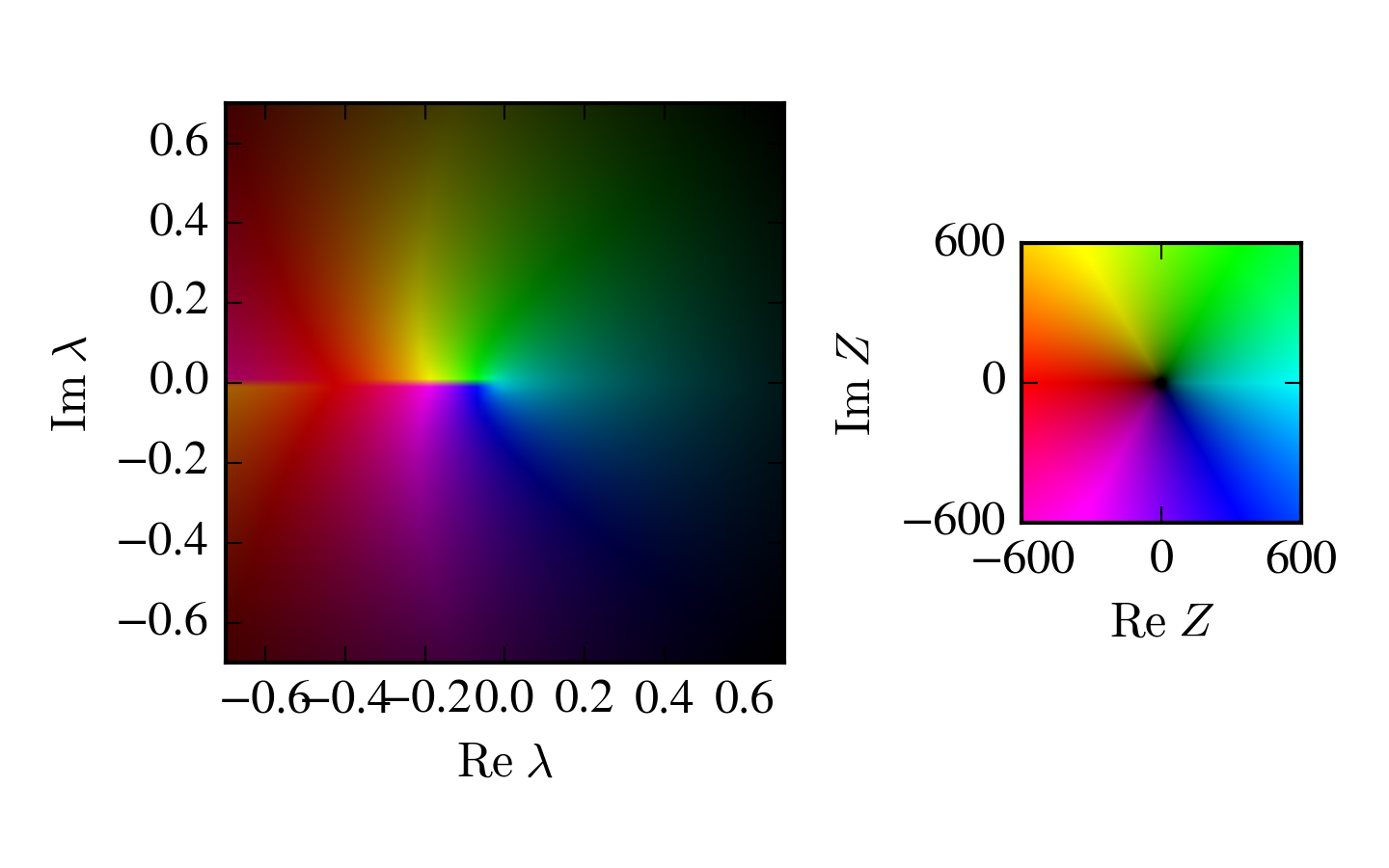# Do Perfect Contours Exist?

Wanted: a manifold such that $\left|\int e^{-S} \;d z\right|=\int \left|e^{-S} \;dz\right|$

This does not automatically solve the sign problem!
• Perfect manifolds might be hard to find
• Hard to sample from
• Observables may have signal-to-noise problem

# Example: One-Dimensional Integrals

$Z = \int dz\;e^{-z^2 - \lambda e^{i\theta} z^4}$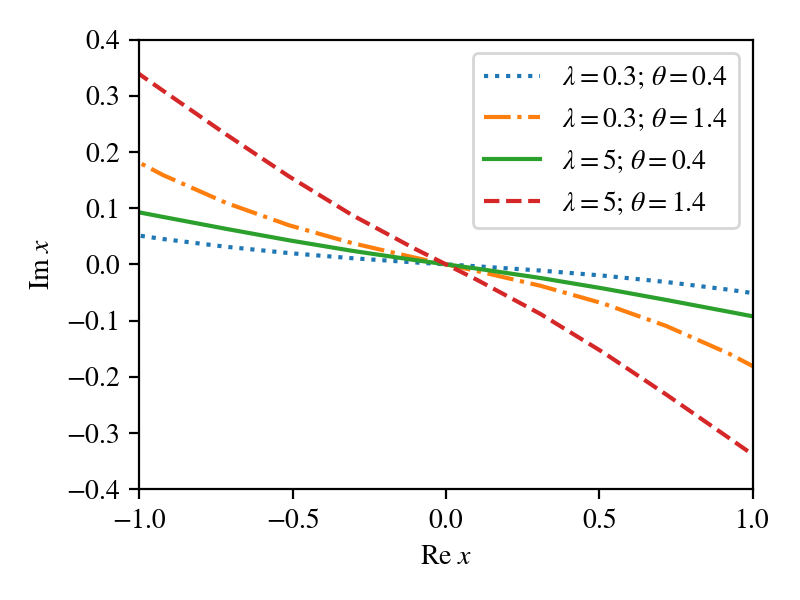# Example: One-Dimensional Integrals

$Z = \int dz\;e^{z^2 - e^{i} z^4 - i z^3}$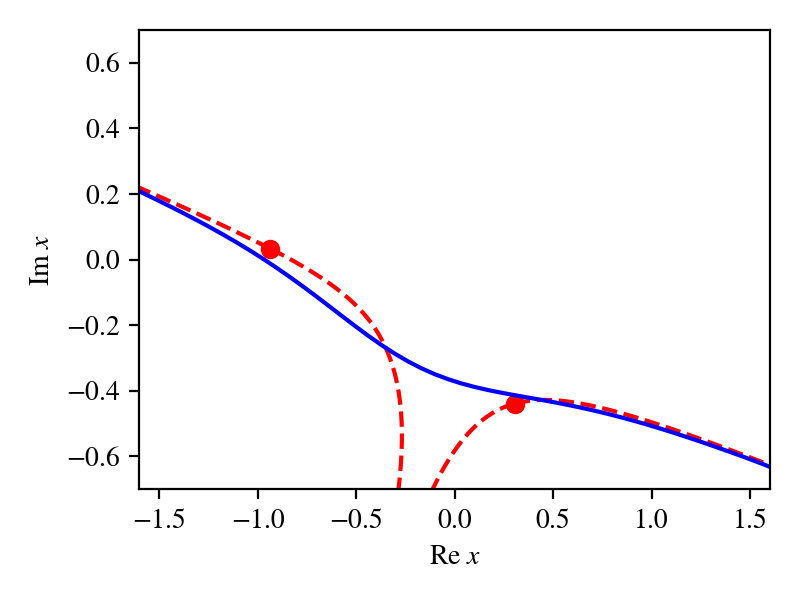# Example With No Perfect Manifold

$Z = \int (\cos \theta + \epsilon) \;d \theta$

$Z = 2 \pi \epsilon$

Quenched partition function is $$O(1)$$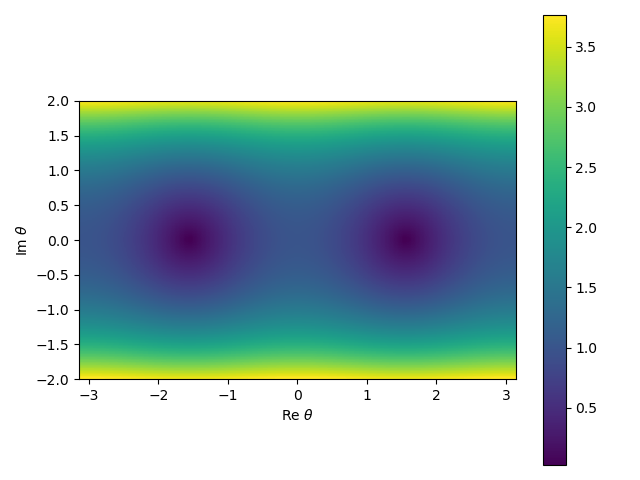Similar methods reveal that no manifold exists for the mean-field Thirring model.

# Directly Modifying the Boltzmann Factor

Modify the partition function by subtracting any function $$g(\phi)$$ that integrates to $$0$$
$Z = \int d\phi\,e^{-S(\phi)} \rightarrow \int d\phi\,\left( e^{-S(\phi)} - g(\phi) \right)$

Physics (the partition function as a function of various sources) is unchanged, but the sign problem...

$Z_Q = \int \left|e^{-S(\phi)}\right| \ne \int \left|e^{-S(\phi)} - g(\phi)\right| = \tilde Z_Q$

All contour deformations are subtractions: $$\int d\phi\, f(\phi) - f(\tilde\phi(\phi)) = 0$$

# Perfect Subtractions Exist

For any sign problem, a subtraction exists that removes it.
$g(\phi) = e^{-S(\phi)} - \frac{\int d\phi\,e^{-S(\phi)}}{\int d\phi}$

This is not unique, but it is "close" to unique. Adding a generic function $$\tilde g \sim e^{-S}$$ breaks the subtraction. Adding a generic function $$\tilde g \sim Z_Q$$ is okay.

General trick to obtain functions that integrate to $$0$$: $$g(\phi) = \frac{\partial}{\partial \phi_i} v_i$$. (Almost useful for machine learning, but $$v \sim e^{-S}$$ is required.)

# Perturbative Subtractions

Any systematic expansion can be used to obtain a subtraction.

Heavy-dense limit: a lattice expansion in large $$\mu$$: $\det K[A] = {\color{blue}2^{-\beta V} e^{\beta V \mu + i \sum_x A_0(x)}} + O(e^{\beta (V-1) \mu})$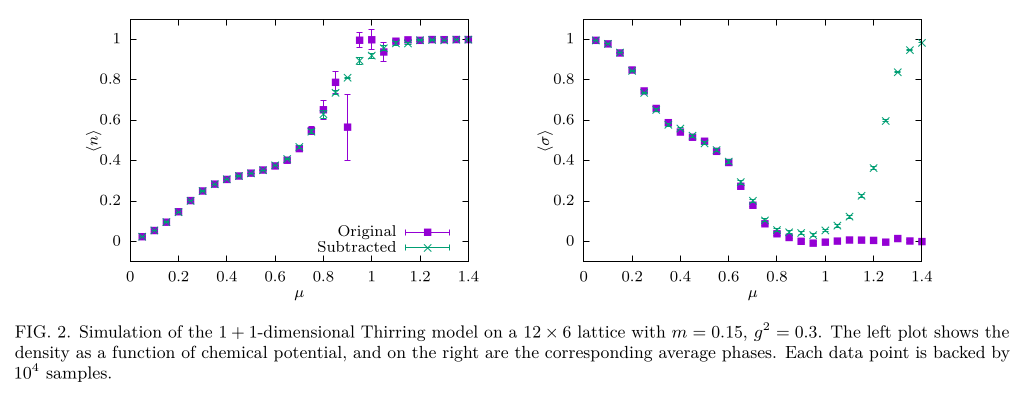# Learning Subtractions

$Z = \int e^{-S} \rightarrow \int e^{-S}\left(1 - v \cdot \nabla S + \nabla \cdot v\right)$

This form of subtraction is the same, at leading order in $$v$$, as performing an infintesimal contour deformation.

$\langle \mathcal O\rangle = \frac{\int e^{-S} \mathcal O}{\int e^{-S}} \rightarrow \frac{\int e^{-S} \left(1 - v \cdot \nabla S + \nabla \cdot v\right) \left(\mathcal O - \frac{v \cdot \nabla \mathcal O}{\left(1 - v \cdot \nabla S + \nabla \cdot v\right) }\right) }{\int e^{-S}\left(1 - v \cdot \nabla S + \nabla \cdot v\right) }$

Other procedures for measuring observables generally result in terrible signal-to-noise.

Training a 2-layer network on a 6-by-6 lattice with $$m_B = 0.33(1)$$, $$m_F=0.35(2)$$: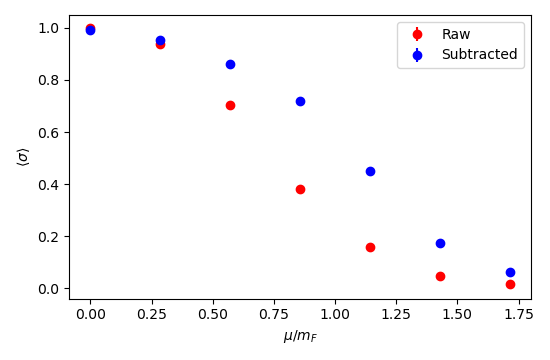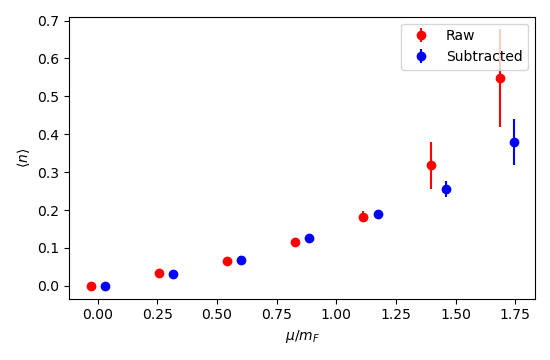Does this trick (meaningfully) undermine the existence guarantees?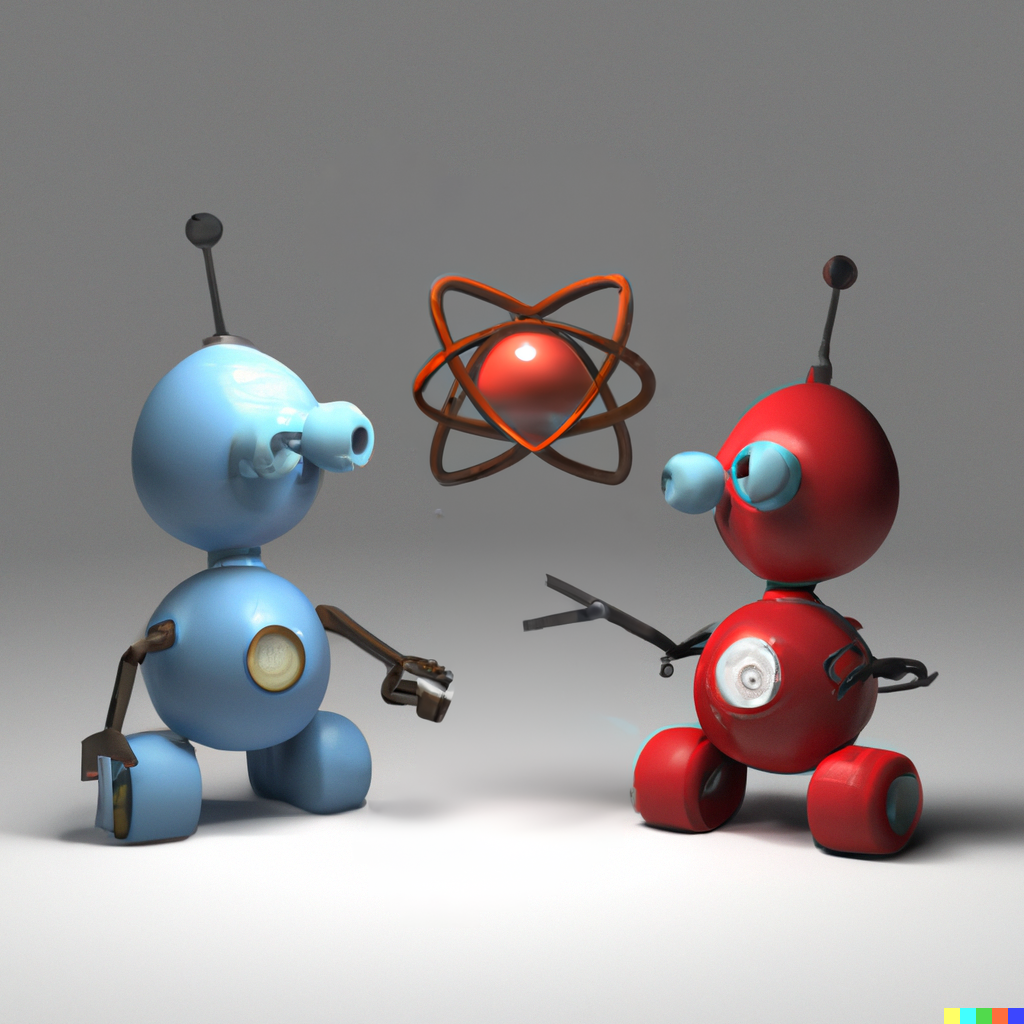/
< >